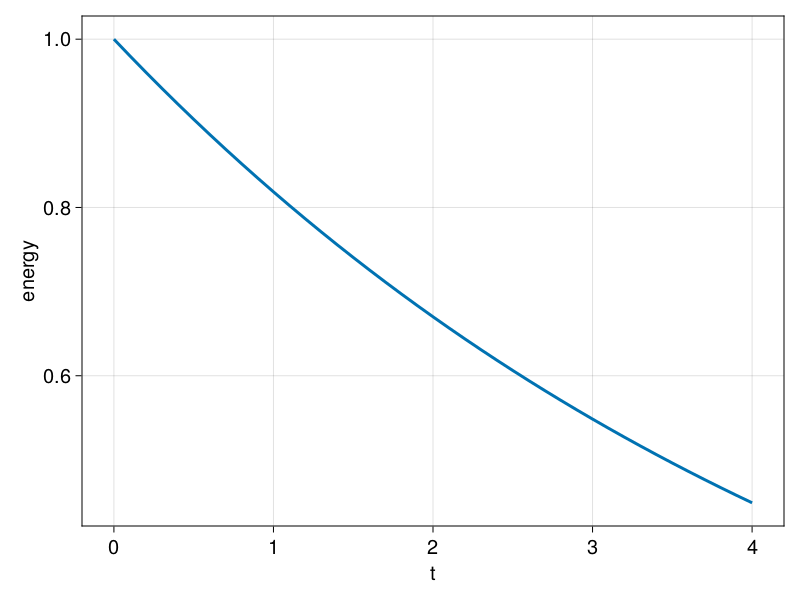# Output

To save output we use Output. Let's see how we can use the example developed in Problem and Diagnostics sections to demonstrate how we can save some output to disk and then load it.

After we have created the problem (prob) and the energy diagnostic (E), we can construct an Output. We first choose where we'd like to output the .jld2 file(s):

filepath = "."
filename = joinpath(filepath, "simplestpde.jld2")
"./simplestpde.jld2"

and the construct the Output. To do so, we provide tuples of fields that we want to output and a function what takes prob as its argument and returns the corresponding value of that field. For this example, let's save the energy E and the state vector sol.

get_uh(prob) = prob.sol

out = Output(prob, filename, (:uh, get_uh), (:E, energy))
Output
├──── prob: FourierFlows.Problem{DataType, Vector{ComplexF64}, Float64, Vector{Float64}}
├──── path: ./simplestpde.jld2
└── fields: Dict{Symbol, Function}(:uh => Main.get_uh, :E => Main.energy)

Note that we haven't saved anything to disk yet!

By calling saveproblem

saveproblem(out)

we write all certain aspects of the problem on the .jld2 file. For example, doing so saves the grid parameters (Lx, nx, ...), everything in prob.params, the the linear operator and its attributes from prob.eqn, and the time-step (prob.clock.dt). All these are very useful in case we'd like to re-create the problem.

Let's open the file and have a quick look what's been written there.

using JLD2
file = jldopen(out.path)
JLDFile /home/runner/work/FourierFlows.jl/FourierFlows.jl/docs/build/simplestpde.jld2 (read-only)
├─📂 eqn
│  ├─🔢 L
│  ├─🔢 dims
│  └─🔢 T
├─📂 clock
│  └─🔢 dt
├─📂 grid
│  ├─🔢 nx
│  └─ ⋯ (2 more entries)
└─📂 params (1 entry)

Accessing the saved values is done using usual HDF5 way, e.g.,

file["grid/nx"]
32

Let's now close the file and move on with our demonstration.

close(file)

Now we can start saving our output fields. We do so via saveoutput, which will go through the list of fields we provided to Output, call the functions that compute the fields, and write their results on disk.

saveoutput(out)

The output fields are saved under "snapshots" group, e.g.,

file = jldopen(out.path)
file["snapshots"]
JLD2.Group
├─📂 t
│  └─🔢 0
├─📂 uh
│  └─🔢 0
└─📂 E
└─🔢 0

Let's close the file again.

close(file)

We can now time-step the problem forward and save the output files on disk every so often.

for _ in 1:40
stepforward!(prob, E, 5)
saveoutput(out)
end

All right! Now we have simulated 200 time-steps saving output every 5 time-steps.

Let's see now how we can load the output we saved in the .jld2 file and visualize it.

We load the .jld2 file and extract the saved iterations.

file = jldopen(out.path)

iterations = parse.(Int, keys(file["snapshots/t"]))
41-element Vector{Int64}:
0
5
10
15
20
25
30
35
40
45
⋮
160
165
170
175
180
185
190
195
200

Then we can construct a vector with all saved times and all saved values for energy.

times = [file["snapshots/t/$iteration"] for iteration in iterations] energies = [file["snapshots/E/$iteration"] for iteration in iterations]

and plot:

using CairoMakie

lines(times, energies; axis = (xlabel = "t", ylabel = "energy"))Lastly, let's load the saved uh fields, process them (get u by convert to physical space), and animate them.

We use Makie's Observable to animate the data. To dive into how Observables work we refer to Makie.jl's Documentation.

using CairoMakie, Printf

nx = file["grid/nx"]
x = file["grid/x"]

n = Observable(1)

u = @lift irfft(file[string("snapshots/uh/", iterations[$n])], nx) title = @lift @sprintf("u(x, t=%1.2f)", file[string("snapshots/t/", iterations[$n])])

fig = Figure()
ax = Axis(fig[1, 1]; xlabel = "x", title)
scatterlines!(ax, x, u; markersize = 14)

frames = 1:length(iterations)

record(fig, "animation.mp4", frames, framerate=16) do i
n[] = i
end
"animation.mp4"